# Continuous lattice

A type of complete lattice, first studied under this name by D.S. Scott [a1], [a12], examples of which occur in many areas of algebra, analysis and topology. Continuous lattices are usually defined in terms of an auxiliary relation, the way-below relation, which is definable in any complete lattice: One says that an elementis way below an element(and writes) if, wheneveris a directed subset ofwith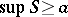, there exists an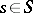with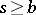. The elements way below a given elementform an ideal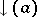(in fact the intersection of all those idealswith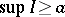); one says thatis continuous if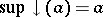for every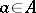. Thus, a continuous lattice is a partially ordered set in which every subset has a least upper bound and in which every element is the least upper bound of all elements way below it. An elementin a complete lattice is compact, or finite (cf. Compact lattice element), if and only if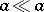; from this it follows that an algebraic lattice is continuous, and in fact continuous lattices may be characterized as those complete lattices which are retracts of algebraic lattices by mappings preserving directed sups. Continuous lattices may also be characterized equationally: they are precisely those complete lattices which satisfy all those instances of the complete distributive law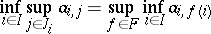(whereis the set of choice functions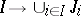) for which the setis (upwards) directed for each. In particular, a completely distributive lattice is continuous; conversely, one can show that ifis a (finitely) distributive complete lattice such that bothandare continuous, thenis completely distributive.

Ifand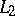are continuous lattices, then the set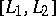of all functions from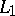topreserving suprema of upwards directed sets is a continuous lattice with respect to the pointwise partial order. The category of continuous lattices and such functions is a Cartesian-closed category (cf. Closed category).

There are two intrinsic topologies which are of importance in the study of continuous lattices. The Scott topology on a complete latticehas for its open sets those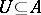which are upper sets (i.e.and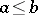implies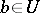) and inaccessible by directed sups (i.e.directed andimplies). A function between complete lattices is continuous for their Scott topologies if and only if it preserves directed sups; thus the Scott-open subsets ofare precisely those whose characteristic functions are Scott-continuous mappings. Equipped with their Scott topologies, continuous lattices are precisely the injective objects (with respect to subspace inclusions, cf. Injective object) in the category of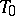topological spaces [a1]; equivalently, they are precisely the retracts of powers of the Sierpiński space (the latter being the two-element latticeequipped with its Scott topology). The second intrinsic topology, the Lawson topology, has as a subbase of open sets the Scott-open sets together with the complements of principal filters (i.e. the sets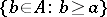,). It is Hausdorff (unlike the Scott topology) and compact, and the binary meet operationis continuous for it; moreover, continuous lattices equipped with their Lawson topologies may be characterized as those compact Hausdorff topological meet semi-lattices for which the continuous semi-lattice of homomorphisms into the unit interval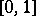separate points (these are sometimes called (compact) Lawson semi-lattices) [a2], [a3]. From this, one obtains yet another, purely lattice-theoretic, characterization of continuous lattices: up to isomorphism, they are precisely the subsets of powers ofwhich are closed under directed sups and arbitrary infs.

In the lattice of all open sets of a topological space, it is easy to see that the relationholds if there is a compact setwith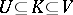; from this it follows that the open-set lattice of a locally compact space (or of a locally quasi-compact space) is continuous. Conversely, the continuous lattices which are (finitely) distributive are exactly the open-set lattices of locally compact topological spaces [a4]. The continuity of the open-set lattices of locally compact spaces is closely related to the good behaviour of function spaces when the domain space is locally compact [a5], [a6].

Iterating a natural morphismand using an inverse limit construction, Scott imbedded any continuous lattice in a continuous lattice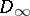which is naturally isomorphic to. Every elementin such a continuous latticemay thus be regarded as a self-map of this domain and vice versa — provided that "self-maps" are morphisms in the category. As a consequence, self-application of the type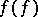is well-defined. Therefore, the continuous latticesare models of the-calculus.

A generalization of the concept of a continuous lattice is that of a continuous poset [a7]. A further generalization, to continuous categories, has also been studied, [a8]. A continuous poset is defined by replacing in the definition of continuous lattice the requirement that all subsets ofhave suprema by the weaker assumption that all non-empty upwards directed sets have suprema and that all sets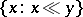are upwards directed.

Continuous posets allow a theory of the type of Pontryagin duality. The lattice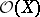of open subsets of a Hausdorff space is a continuous lattice if and only ifis locally compact. In this case its Pontryagin dual is the set of compact subsets with reversed inclusion as order relation.

A domain is a continuous poset in which, first, every element is the supremum of compact elements, secondly, there are countably many compact elements, and, finally, every set which has an upper bound has a supremum. Domains have applications in the semantics of high-level programming languages; introductions into this aspect can be found in [a14] and [a15].

A detailed account of continuous lattice theory is given in [a9], and a more concise one in [a10], Chapt. 7. Reference [a11] discusses some more recent developments in the subject and contains an extensive bibliography on continuous lattices up to 1985.

Continuous lattices were introduced by Dana S. Scott [a1], [a12] as a basis for an abstract theory of computation by which a "computation" is approximated better and better by "finite approximations" .

Continuous lattices should not be confused with continuous geometries, which are complete lattices of an entirely different type. Cf. Orthomodular lattice for the notion of continuous geometry.

How to Cite This Entry:
Continuous lattice. Encyclopedia of Mathematics. URL: http://encyclopediaofmath.org/index.php?title=Continuous_lattice&oldid=51759